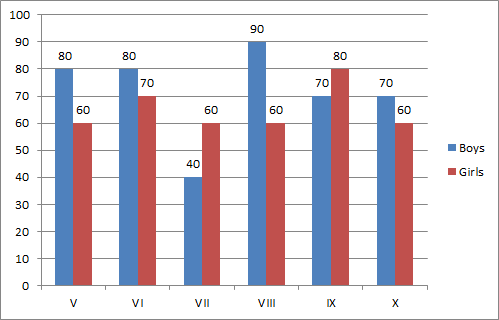# Numerical Reasoning - Test 10

Instructions:

Study the following graph and answer the questions 1-41:

In which standard is the difference between the results of girls and boys maximum?

 A. V B. VII C. X D. VIII Answer Report Discuss Option: D Explanation : The difference between the results of girls and boys in V standard is 20; in VI standard is 10; in VII standard is 20 and in VIII standard is 30; in IX standard is 10 and  in X standard is 10. So, it is maximum in VIII standard. Click on Discuss to view users comments. Write your comments here:
2:

In which standard is the result of boys less than the average result of the girls?

 A. VII B. IX C. VI D. VIII Answer Report Discuss Option: A Explanation : Average result of girls. = (60+70+60+60+80+60)/6 = 390/6 =65% Click on Discuss to view users comments. Write your comments here:
3:

In which pair of standards are the results of the girls and boys in inverse proportion?

 A. V & X B. VI and VIII C. V & IX D. VI & IX Answer Report Discuss Option: D Explanation : In VI standard, the results of boys and girls are in the ratio 8:7 while in IX standard, the results of boys and girls are in the ration 7: 8. Click on Discuss to view users comments. Write your comments here:
4:

In which standard is the result of the girls more than the average result of the boys for the school?

 A. XI B. VIII C. VI D. X Answer Report Discuss Option: A Explanation : Average result of boys = 1/6 (80+80+40+90+70+70)  = 430/6 = 71.7% Clearly, in IX standard the result of girls is more than the average result of the boys.   Click on Discuss to view users comments. Write your comments here:

## Suggest an improvement

1. Take a free Numerical test before your next interview.
2. Take a numeracy psychometric test designed especially for CES and USB
3. Here you can practice questions for ceb numerical reasoning test.
4. These questions can be used for investment banking numerical test .
5. Here you will find questions answers for numeracy psychometric test.
6. This section provides you free online psychometric test.
7. These questions can be used for numerical assessment